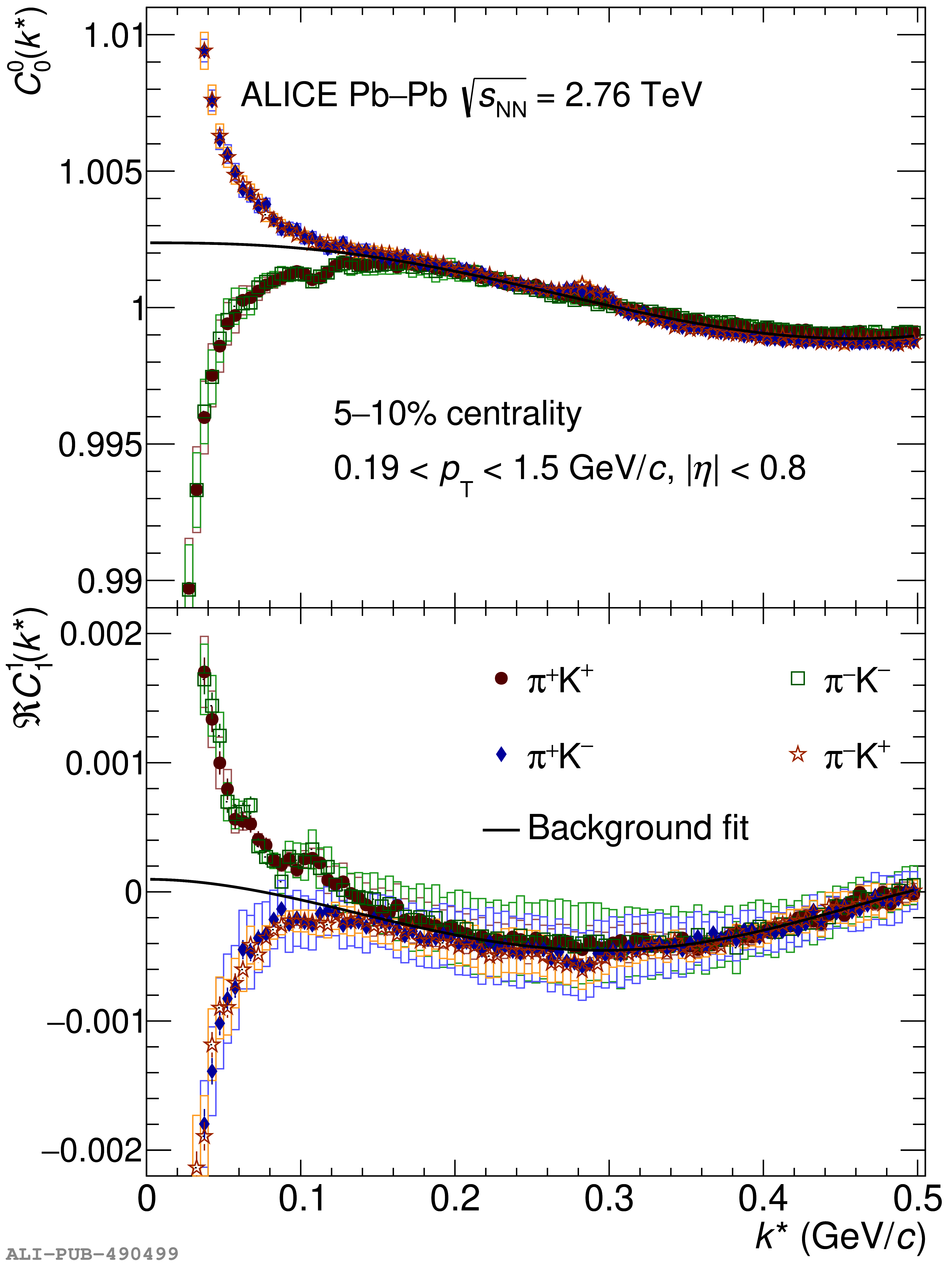# Figure 2

 The $C^{0}_{0}$ (top panel) and $\Re C^{1}_{1}$ (botton panel) components of the pion--kaon correlation functions in the 5--10\% centrality class showing the non-femtoscopic background in the spherical-harmonic representation, positive field polarity. The background fit corresponds to a 6$^{\text{th}}$ order polynomial function common for all charge combinations. The two structures visible in the correlation function at 0.11 GeV$/c$ and at 0.29 GeV$/c$ correspond to the remaining effect from track merging and the K$^{*}$ resonance, respectively. The statistical and systematic uncertainties are shown as vertical bars and boxes, respectively.